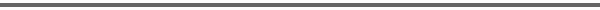Journal Home Page Cumulative Index List of all Volumes Complete Contentsof this Volume Previous Article Next Article Journal of Convex Analysis 11 (2004), No. 2, 401--411 Copyright Heldermann Verlag 2004A Necessary and Sufficient Optimality Condition for a Class of Nonconvex Scalar Variational Problems Guillaume Carlier Universite Bordeaux IV, GRAPE, UMR CNRS 5113, Avenue Leon Duguit, 33608 Pessac, France, Guillaume.Carlier@math.u-bordeaux.fr[Abstract-pdf] This article studies the minimization of the functional $$u\mapsto\int_{0}^{1}f(\dot{u})$$ among all convex functions $u$ that satisfy the additional obstacle constraint $u\geq \ovu$, $u(0)=\ovu(0)$, $u(1)=\ovu(1)$ where $\ovu$ is a given convex function. We first show that this nonconvex problem is in fact equivalent to a linear programming problem. This enables us to establish a necessary and sufficient optimality condition. Keywords: convexity constraint, monotone rearrangements, duality. FullText-pdf (271 KB) for subscribers only.